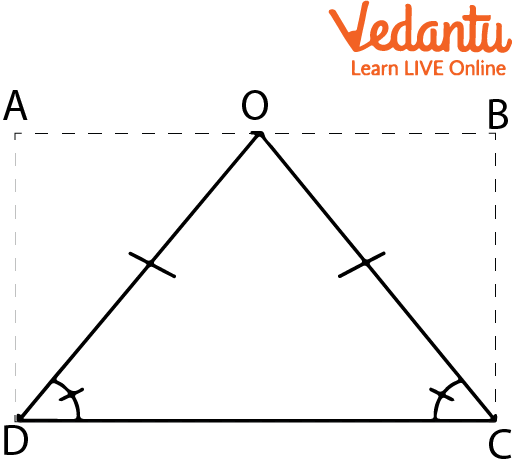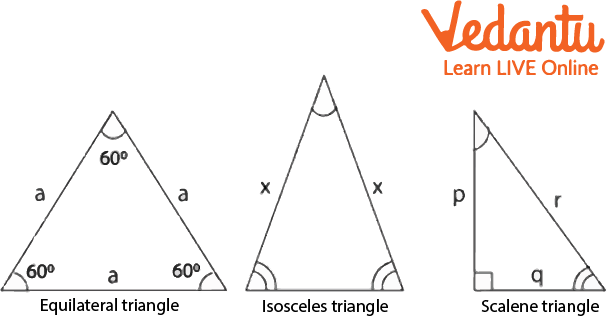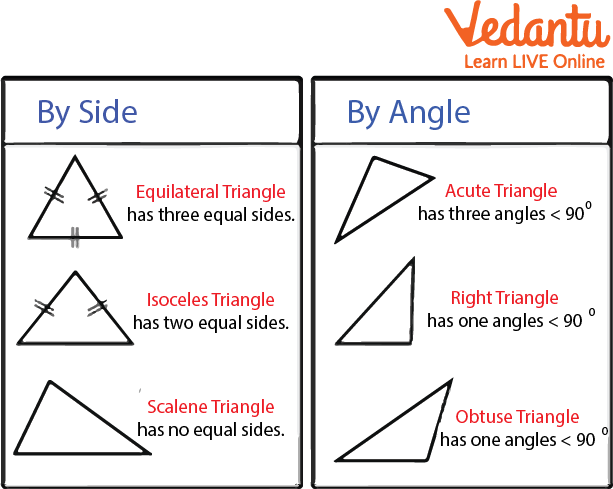Courses
Courses for Kids
Free study material
Offline Centres
More

# Base of an Isosceles TriangleLast updated date: 01st Dec 2023
Total views: 101.4k
Views today: 1.01k## What is an Isosceles Triangle?

Triangles classified as isosceles have at least two sides with equal sides or angles. As three-sided unconstrained polygons, triangles are known to exist. Based on the length of their sides, triangles are called equilateral or isosceles.

No matter which way the triangle's peak (or apex) points, an isosceles triangle always has two equal sides. The following are some tips on isosceles triangles:

• There are equal sides to it. The basis angles are two equal angles that make up the object.

• A right isosceles triangle is one where the third angle is 90 degrees.

As we can see, the given figure $OD = OC$ and $\angle D$ are equal to $\angle C$, so we can say that this given triangle is an isosceles triangle.Isosceles Triangle

## Properties of an Isosceles Triangle

The properties of the isosceles triangle are given below:

• Two equal sides and two equal angles.

• The two equal sides of an isosceles triangle are called the legs and the angle between them is called the vertex or apex angle.

• The side opposite the vertex angle is called the base and base angles are equal.

• The perpendicular from the apex angle bisects the base and the apex angle.

• The perpendicular drawn from the apex angle divides the isosceles triangle into two congruent triangles and is also known as its line of symmetry.

## Isosceles Triangle DiagramThree Types of Triangles

In the above figure, we can find the visual difference between the three different types of triangles to compare with an isosceles triangle. The first figure is of an equilateral triangle and second is an isosceles triangle and the last one is Scalene.Different Triangles

In the above-given figure, we can see many types of triangles. All triangles given above state their features on their own. First three have their properties by sides, and the remaining three by their angles. Each triangle has its features and properties. As we can see in the diagram that equilateral triangle has three equal angles, the isosceles have two equal angles, and the scalene has no equal angles. Similarly, the acute triangle has three angles less than 90, and the right has only one angle equal to 90, and the obtuse has an angle of more than 90.

## The Base of an Isosceles Triangle

A triangle with two equal-length sides is termed an isosceles triangle. The base refers to the triangle's third side. The angle between the legs is known as the vertex angle. The base angles are those angles that have the base as one of their sides.

Q. Given an isosceles triangle measure of the unequal angle is 70° and the other two equal angles measure x; then what is the value of x?

Ans: The given angle is 70.

${\bf{70}}^\circ {\rm{ }} + {\rm{ }}{\bf{x}}{\rm{ }} + {\rm{ }}{\bf{x}}{\rm{ }} = {\rm{ }}{\bf{180}}^\circ$

${\bf{70}}^\circ {\rm{ }} + {\rm{ }}{\bf{2x}}{\rm{ }} = {\rm{ }}{\bf{180}}^\circ$

${\bf{2x}}{\rm{ }} = {\rm{ }}{\bf{180}}{\rm{ }}-{\rm{ }}{\bf{70}}{\rm{ }} = {\rm{ }}{\bf{110}}^\circ$

${\bf{x}}{\rm{ }} = {\rm{ }}\frac{{110}}{2}{\rm{ }} = {\rm{ }}{\bf{55}}^\circ$

Hence, The value of x is 55.

## Area of an Isosceles Triangle

The region that an isosceles triangle occupies in two dimensions is referred to as its area. Typically, the base and height of an isosceles triangle are divided by two to create the triangle. The following equation can be used to determine an isosceles triangle's area:

An isosceles triangle's area is A = ½* b* h square units.

## Examples

Example 1: Calculate the area of an isosceles triangle given $b{\rm{ }} = {\rm{ }}12{\rm{ }}cm$ and $h{\rm{ }} = {\rm{ }}17{\rm{ }}cm$?
Ans:

Given , $b{\rm{ }} = {\rm{ }}12{\rm{ }}cm$

$h{\rm{ }} = {\rm{ }}17{\rm{ }}cm$

Area of Isosceles Triangle $= {\rm{ }}\left( {1/2} \right){\rm{ }} \times {\rm{ }}b{\rm{ }} \times {\rm{ }}h$

$= {\rm{ }}\left( {1/2} \right){\rm{ }} \times {\rm{ }}12{\rm{ }} \times {\rm{ }}17$

$= {\rm{ }}6{\rm{ }} \times {\rm{ }}17$

$= {\rm{ }}102{\rm{ }}c{m^2}$

Example 2: Find the length of the base of an isosceles triangle whose area is$243{\rm{ }}c{m^2}$, and the altitude of the triangle is $27{\rm{ }}cm.$

Ans: Area of the triangle is $243{\rm{ }}c{m^2}$

Height of the triangle is $27{\rm{ }}cm$

The base of the triangle = b =?

Area of Isosceles Triangle is $\left( {1/2} \right){\rm{ }} \times {\rm{ }}b{\rm{ }} \times {\rm{ }}h$

$243{\rm{ }} = {\rm{ }}\left( {1/2} \right){\rm{ }} \times {\rm{ }}b{\rm{ }} \times {\rm{ }}27$

$243{\rm{ }} = {\rm{ }}\left( {b \times 27} \right)/2$

$b{\rm{ }} = {\rm{ }}\left( {243 \times 2} \right)/27$

$b{\rm{ }} = {\rm{ }}18{\rm{ }}cm$

Hence, the base of the triangle is 18 cm.

## Summary

In this article, we have covered various topics regarding isosceles triangles. We understood that the basic properties of the triangle are that both the sides and angles are equal. We have also solved the examples and solved questions to understand them better.

## FAQs on Base of an Isosceles Triangle

1. What is the difference between the isosceles triangle and the isosceles right angle triangle?

A triangle with at least two equal sides is said to be isosceles. Due to the equality of the triangle's two legs, the corresponding angles are also equal. The isosceles right triangle has two equal sides and one angle that is exactly 90 degrees.

2. What do you mean by the area of an isosceles triangle?

The area of an isosceles triangle is nothing but the area occupied by the triangle.

3. What is the angle distribution of the right isosceles triangle?

The right isosceles triangle has one 90-degree and two 45-degree angles.